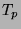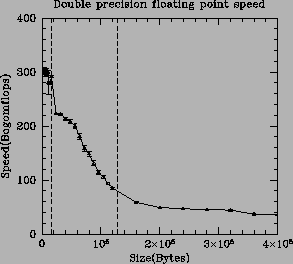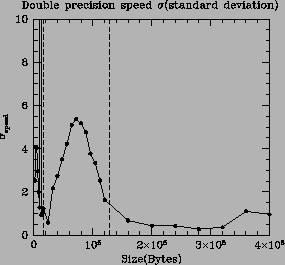Next: Conclusions Up: Rates, Latencies and Bandwidths Previous: Netperf Results   Contents

## CPU Results

The CPU numerical performance is one of the most difficult components to precisely quantify. On the one hand, peak numerical performance is a measure always published by the vendor. On the other hand, this peak is basically never seen in practice and is routinely discounted.

CPU performance is known to be heavily dependent on just what the CPU does, the order in which it does it, the size and structure and bandwidths and latencies of its various memory subsystems including L1 and L2 caches, and the way the operating system manages cached pages. This dependence is extremely complex and studying one measure of performance for a particular set of parameters is not very illuminating if not misleading. In order to get any kind of feel at all for real world numerical performance, floating point instruction rates have to be determined for whole sweeps of e.g. accessed vector memory lengths.

What this boils down to is that there is very little numerical code that is truly typical'' and that it can be quite difficult to assign a single rate to floating point operations like addition, subtraction, multiplication, and division that might not be off by a factor of five or more relative to the rate that these operations are performed in your code. This translates into large uncertainties and variability of, for example,with parallel program scale and design.

Still, it is unquestionably true that a detailed knowledge of the MFLOPS'' (millions of floating point operation per second) that can be performed in an inner loop of a calculation is important to code and beowulf design. Because of the high dimensionality of the variables upon which the rate depends (and the fact that we perforce must project onto a subspace of those variables to get any kind of performance picture at all) the resulting rate is somewhat bogus but not without it uses, provided that the tool used to generate it permits the exploration of at least a few of the relevant dimensions that affect numerical performance. Perhaps the most important of these are the various memory subsystems.To explore raw numerical performance the cpu-rate benchmark is used [cpu-rate]. This benchmark times a simple arithmetic loop over a vector of a given input length, correcting for the time required to execute the empty loop alone. The operations it executes are:

x[i] = (1.0 + x[i])*(1.5 - x[i])/x[i];

where x[i] is initialized to be 1.0 and should end up equal to 1.0 (within any system roundoff error) afterwards as well.

Each execution of this line counts as four floating point operations'' (one of each type, where x[i] might be single or double precision) and by counting and timing one can convert this into FLOPS. As noted, the FLOPS it returns are somewhat bogus - they average over all four arithmetic operations (which may have very different rates), they contain a small amount of addressing arithmetic (to access the x[i] in the first place) that is ignored, they execute in a given order which may or many not accidentally benefit from floating point instruction pipelining in a given CPU, they presuming streaming access of the operational vector.

Still, this is more or less what what I think most people'' would mean when they ask how fast a system can do floating point arithmetic in the context of a loop over a vector. We'll remind ourselves that the results are bogus by labeling them BOGOflops''.

These rates will be largest when both the loop itself and the data it is working on are already on the CPU'' in registers, but for most practical purposes this rarely occurs in a core loop in compiled code that isn't hand built and tuned. The fastest rates one is likely to see in real life occur when the data (and hopefully the code) live in L1 cache, just outside the CPU registers. lmbench contains tests which determine at least the size of the L1 data cache size and its latency. In the case of lucifer, the L1 size is known to be 16 KB and its latency is found by lmbench to be 6 nanoseconds (or roughly 2-3 CPU clocks).

However, compiled code will rarely will fit into such a small cache unless it is specially written to do so. In any event we'd like to see what happens to the floating point speed as the length of the x[i] vector is systematically increased. Note that this measurement combines the raw numerical rate on the CPU with the effective rate that results when accounting for all the various latencies and bandwidths of the memory subsystem. Such a sequence of speeds as a function of vector lengths is graphed in figure 9.2.

This figure clearly shows that double precision floating point rates vary by almost an order of magnitude as the vector being operated on stretches from wholly within the L1 cache to several times the size of the L2 cache. Note also that the access pattern associated with the vector arithmetic is the most favorable one for efficient cache operation - sequential access of each vector element in turn. The factor of about seven difference in the execution speeds as the size of this vector is varied has profound implications for both serial code design and parallel code design. For example, the whole purpose of the ATLAS project [ATLAS] is to exploit the tremendous speed differential revealed in the figure by optimally blocking problems into in-cache loops when doing linear algebra operations numerically.

There is one more interesting feature that can be observed in this result. Because linux on Intel lacks page coloring, there is a large variability of numerical speeds observed between runs at a given vector size depending on just what pages happen to be loaded into cache when the run begins. In figure 9.3 the variability (standard deviation) of a large number (100) of independent runs of the cpu-rate benchmark is plotted as a function of vector size. One can easily pick out the the L1 and L2 cache boundaries as they neatly bracket the smooth peak visible in this figure. Although the L1 cache boundary is simple to determine directly from tests of memory speed, the L2 cache boundary has proven difficult to directly observe in benchmarks because of this variability. This is a new and somewhat exciting result - L2 boundaries can be revealed by a susceptibility'' of the underlying rate.Next: Conclusions Up: Rates, Latencies and Bandwidths Previous: Netperf Results   Contents
Robert G. Brown 2004-05-24